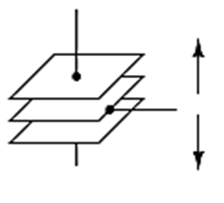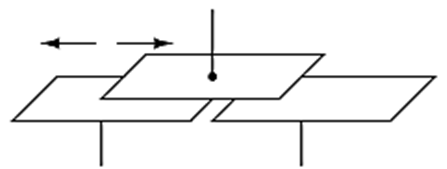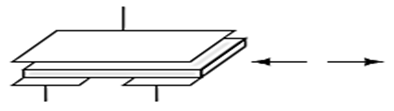### Scales

May 02, 2016

#### by Tom Lish

Whether you are an engineer or a consumer, many daily applications involve capacitance. Capacitance can be measured using a capacitor, however it is not as simple as that. There are three different methods in which the capacitor measures capacitance.

1.Displacement of Parallel Plates

• Diaphragm deflections is simplest way to changethe measure of capacitance, by varying the distance between electrode plates
• This method measures the amount of energy that can be stored by the slight amount one electrode plate of the diaphragm deflects in response to changes in applied force
• The diaphragm can deflect inwards or outwards (opening/closing gap)
• Fun Fact: Setra uses this method by converting the change in capacitance into a linear analog output (voltage/current) for our pressure transducers

2. Change in Area- Shift in Electrode Plates• Measures capacitance between two moving electrode plates
• As the plates move in separate directions, in respect to one another, the capacitance between the plates decreases

3. Change in Dielectric Material

• Dielectric material is an insulating layerbetween the two conducting electrode plates
• Dielectric material examples include solids and gases
• Can be used with displacement parallel plates or change in area